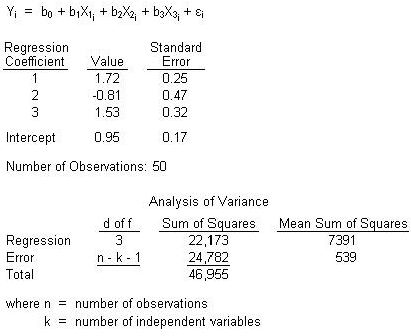### CFA Practice Question

There are 208 practice questions for this study session.

### CFA Practice Question

An analyst is currently reviewing the following regression analysis:At a significance level of 5%, which of the following statements would best reflect the significance of the regression coefficients together as a whole?

A. The calculated F-statistic is greater than the critical F-statistic of 7.25, thus making the model significant.
B. The calculated F-statistic of 13.71 is inside the bound of the acceptance range, thus making the model insignificant.
C. The calculated F-statistic of 13.71 is outside the bound of the acceptance range, thus making the model significant.
Explanation: 1. Set up the test (F-test):
H0: b1 = b2 = b3 = 0.
Hb1: At least one of the coefficients does not equal to 0.

2. Compute the 'Calculated F': Fcalc = MSSR/MSSE = 7391/539 = 13.71.

3. Compute the 'Critical F'. Fcalc at the 5% significance level with a d of f(MSSR) = 3 (numerator) and d of f(MSSE) = 46 (denominator) equals: (approximately) 2.81.

4. Decision: since the calculated F statistics is greater than the critical value, the null hypothesis is rejected. Thus, at least one of the coefficients does not equal 0, giving the regression equation significance.